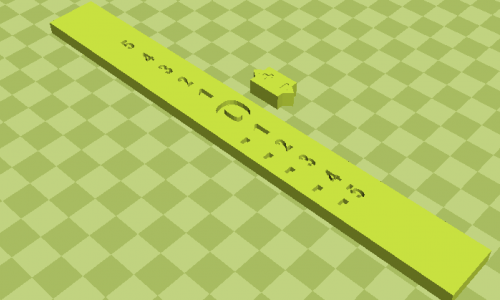# “The Elevator”My project will be a number line that consists of positive and negative numbers. The number line will be vertical instead of horizontal to act as an elevator chute. There will be another piece that can slide up or down according to the problem that the student is working on. This extra piece of the manipulative will be considered the “elevator.” The idea of this number line is to help students physically picture how to add and subtract positive and negative numbers.  It is easier for students to understand concepts in math when they can relate to the content. This is why I chose to use an elevator, because I can assume that most of the students have at some point come in contact with an elevator in their lives. On the elevator there will be symbols of up and down arrows and a positive and negative sign so that the students can determine which way the elevator should go according to the problem. The 0 on the number line represents the ground level. All the positive numbers represent different floors above the ground level, and all the negative numbers represent different floors below ground level, or in the basement.

When the student uses this manipulative, they can physically move the “elevator” up and down the floors. For an addition problem such as, -5+2, the student will start the “elevator” at the mark where it says -5. This indicates that it is 5 levels under ground level. Since the problem asks the student to add 2, the elevator will move up 2 “floors” and the student will see that the answer is -3.

As a student I struggled with learning how to add and subtract negative numbers. It was a concept that I always needed to draw a number line and count on the number line to solve. What confused me the most was when adding to a negative number it was hard for me to conceive that the actual number would be a smaller digit. For example -6 + 3, I would always solve it to be -9 because adding meant bigger numbers so, -3 as the answer didn’t make sense to me. My background in learning how to add and subtract negative numbers is why I came up with this idea. Having the number line be a vertical manipulative and reference an elevator explains why when you are adding to a negative number, you need to go up towards the ground level. This will result in a “smaller” digit but of course the value of this digit is bigger, because it is “higher ground”.

Children use direct modeling to solve problems that may be more difficult to them. They are able to take the problem step by step and get to the answer. This manipulative will do exactly that. Each problem, the student will start on the number line with the first digit given, and depending on what is being asked they will move the “elevator” in that direction. If this project were to fail I would try using a different concept for the number line. Instead of using an elevator chute, I could make reference to something else they may be learning in a different subject. For example, if they are learning about the different layers of Earth and the atmosphere in Science class, I could make each level pertain to each of those layers.

Some testing that I would carry out for this manipulative is to give them worksheets of problems that they would be able to use this number line. After enough practice, I could quiz them on adding and subtracting negative numbers without the manipulative to see if the idea of negative numbers sticks. Fortunately, it is very easy for a student to draw this manipulative on a piece of paper in the event that they do not have the actual manipulative to use. If they become stuck on a problem, they simply have to create a vertical number line and count the correct amount of “floors” up or down. The hope is they will not have to physically see the number line, but can reference an elevator in their minds, and associate the numbers with different floors in a building to come up with the right answer.